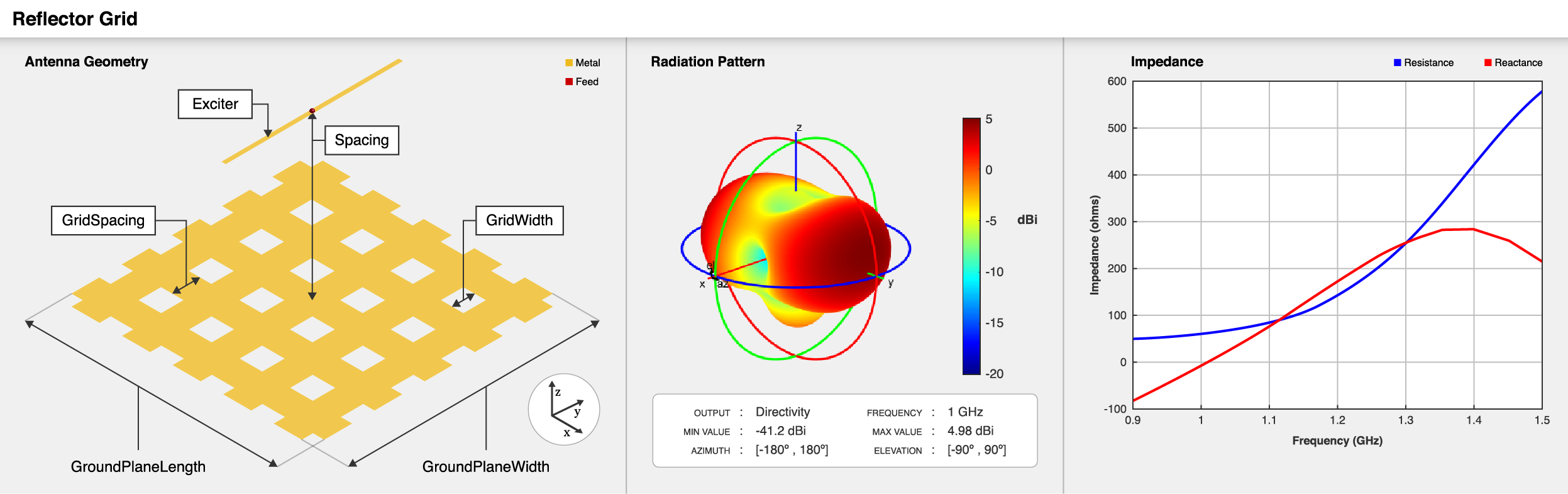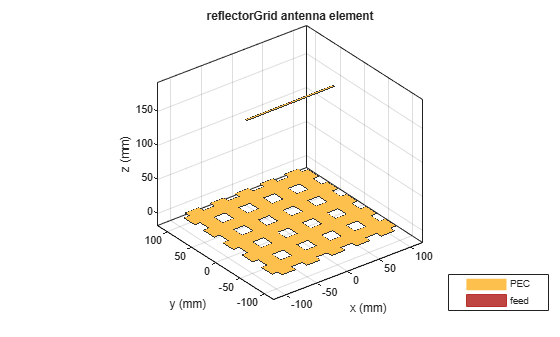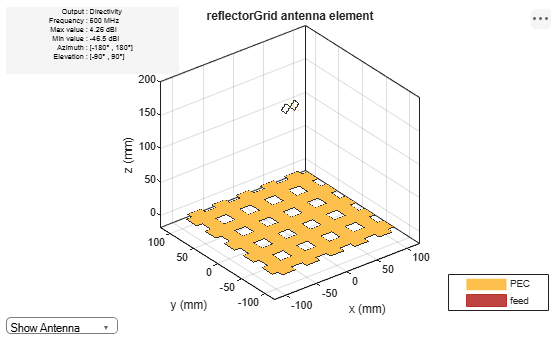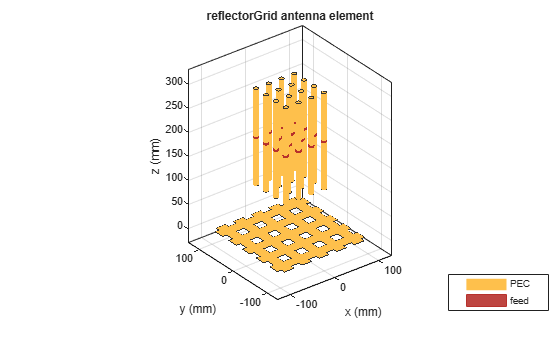Main Content

# reflectorGrid

Create grid reflector-backed antenna

## Description

The `reflectorGrid` object creates a grid reflector-backed antenna. The grid reflector uses a grid of parallel wires or bars oriented in one direction. Grid reflectors can be used as high-gain antennas in point-to-point communications.## Creation

### Syntax

``ant = reflectorGrid``
``ant = reflectorGrid(Name,Value)``

### Description

example

````ant = reflectorGrid` creates a grid reflector-backed antenna. The default antenna object has an exciter as a dipole with the feed point located at the origin on the X-Y plane, and the antenna dimensions are chosen for an operating frequency of 1 GHz.```

example

````ant = reflectorGrid(Name,Value)` sets Properties using name-value pairs. For example, `reflectorGrid('GroundPlaneWidth',0.6)` creates a grid reflector with a width of 0.6 meters. You can specify multiple name-value pairs. Enclose each property name in quotes. Properties not specified retain their default values. ```

## Properties

expand all

Antenna type used as an exciter, specified as any single-element antenna object. Except reflector and cavity antenna elements, you can use any of the antenna elements or array elements in the Antenna Toolbox™ as an exciter.

Example: `'Exciter',horn`

Example: `ant.Exciter = horn`

Example: ```ant.Exciter = linearArray('patchMicrostrip')```

Distance between reflector and exciter, specified as a positive scalar in meters.

Example: `'Spacing',0.259`

Example: `ant.Spacing = 0.195`

Data Types: `double`

Reflector length along the X-axis, specified as a positive scalar in meters.

Example: `'GroundPlaneLength',0.6`

Example: `ant.GroundPlaneLength = 0.18`

Data Types: `double`

Reflector width along the Y-axis, specified as a positive scalar in meters.

Example: `'GroundPlaneWidth',0.6`

Example: `ant.GroundPlaneWidth = 0.18`

Data Types: `double`

Type of the grid used in the reflector, specified as either one of the following:

• `'H'` — grids are arranged horizontally in the reflector.

• `'V'` — grids are arranged vertically in the reflector.

• `'HV'` or `'VH'` — grids are arranged both horizontally and vertically in the reflector.

Example: `'GridType','H'`

Example: `ant.GridType = 'V'`

Data Types: `char`

Distance between the two grid cells, specified as a positive scalar in meters.

Example: `'GridSpacing',0.018`

Example: `ant.GridSpacing = 0.014`

Data Types: `double`

Width of each grid cell, specified as a positive scalar in meters.

Example: `'GridWidth',0.3`

Example: `ant.GridWidth = 0.28`

Data Types: `double`

Type of the metal used as a conductor, specified as a metal material object. You can choose any metal from the `MetalCatalog` or specify a metal of your choice. For more information, see `metal`. For more information on metal conductor meshing, see Meshing.

Example: `m = metal('Copper'); 'Conductor',m`

Example: `m = metal('Copper'); ant.Conductor = m`

Tilt angle of the antenna, specified as a scalar or vector with each element unit in degrees. For more information, see Rotate Antennas and Arrays.

Example: `'Tilt',90`

Example: `ant.Tilt = 90`

Example: `'Tilt',[90 90]`,`'TiltAxis',[0 1 0;0 1 1]` tilts the antenna at 90 degrees about the two axes defined by the vectors.

Note

The `wireStack` antenna object only accepts the dot method to change its properties.

Data Types: `double`

Tilt axis of the antenna, specified as:

• Three-element vector of Cartesian coordinates in meters. In this case, each coordinate in the vector starts at the origin and lies along the specified points on the X-, Y-, and Z-axes.

• Two points in space, each specified as three-element vectors of Cartesian coordinates. In this case, the antenna rotates around the line joining the two points in space.

• A string input describing simple rotations around one of the principal axes, 'X', 'Y', or 'Z'.

For more information, see Rotate Antennas and Arrays.

Example: `'TiltAxis',[0 1 0]`

Example: `'TiltAxis',[0 0 0;0 1 0]`

Example: `ant.TiltAxis = 'Z'`

Note

The `wireStack` antenna object only accepts the dot method to change its properties.

Data Types: `double`

Lumped elements added to the antenna feed, specified as a `lumpedElement` object. You can add a load anywhere on the surface of the antenna. By default, the load is at the feed. For more information, see `lumpedElement`.

Example: `'Load',lumpedelements`, where `lumpedelements` is the load added to the antenna feed.

Example: `ant.Load = lumpedElement('Impedance',75)`

## Object Functions

 `show` Display antenna or array structure; display shape as filled patch `impedance` Input impedance of antenna; scan impedance of array `sparameters` S-parameter object `returnLoss` Return loss of antenna; scan return loss of array `vswr` Voltage standing wave ratio of antenna `pattern` Radiation pattern and phase of antenna or array; Embedded pattern of antenna element in array `patternAzimuth` Azimuth pattern of antenna or array `patternElevation` Elevation pattern of antenna or array `axialRatio` Axial ratio of antenna `beamwidth` Beamwidth of antenna `current` Current distribution on metal or dielectric antenna or array surface `charge` Charge distribution on metal or dielectric antenna or array surface `efficiency` Radiation efficiency of antenna `EHfields` Electric and magnetic fields of antennas; Embedded electric and magnetic fields of antenna element in arrays `mesh` Mesh properties of metal or dielectric antenna or array structure `optimize` Optimize antenna or array using SADEA optimizer `design` Design prototype antenna or arrays for resonance at specified frequency `rcs` Calculate and plot radar cross section (RCS) of platform, antenna, or array `numGridsToSpacing` Calculate grid spacing in grid for `reflectorGrid` object

## Examples

collapse all

Create and view a grid reflector-backed antenna object with default properties.

```ant = reflectorGrid; show(ant)```Plot the radiation pattern of the antenna at 1 GHz.

`pattern(ant,1e9)`Create and view a grid reflector-backed biquad antenna with an arm length of 0.01 meters.

```d = biquad('ArmLength',0.01); h = reflectorGrid('Exciter',d); show(h)```Plot the radiation pattern of the antenna at 0.6 GHz.

`pattern(h,0.6e9)`Create and view grid reflector-baked dipole blade antenna.

```d = dipoleBlade('Length',0.1,'TaperLength',0.05,'FeedGap',0.002); h = reflectorGrid('Exciter',d); show(h)```Change the grid type from `'HV'` to `'H'`.

`h.GridType = 'H';`

View the antenna with grid type `'H'`.

`show(h)`Plot the radiation pattern at 1 GHz.

`pattern(h,1e9)`Create a rectangular array of cylindrical dipole antennas.

```d = dipoleCylindrical('Length',0.2,'Radius',0.005); arr = rectangularArray('Element',d,'Size',[4 4],'RowSpacing',0.029,'ColumnSpacing',0.029);```

Create a grid reflector-backed rectangular array.

`ant = reflectorGrid('Exciter',arr,'Spacing',0.2)`
```ant = reflectorGrid with properties: Exciter: [1x1 rectangularArray] Spacing: 0.2000 GroundPlaneLength: 0.2000 GroundPlaneWidth: 0.2000 GridType: 'HV' GridSpacing: 0.0180 GridWidth: 0.0220 Conductor: [1x1 metal] Tilt: 0 TiltAxis: [1 0 0] Load: [1x1 lumpedElement] ```
`show(ant)`Balanis, Constantine A. Antenna Theory: Analysis and Design. 3rd ed. Hoboken, NJ: John Wiley, 2005.

## Support

#### Hybrid Beamforming for Massive MIMO Phased Array Systems

Download the white paper